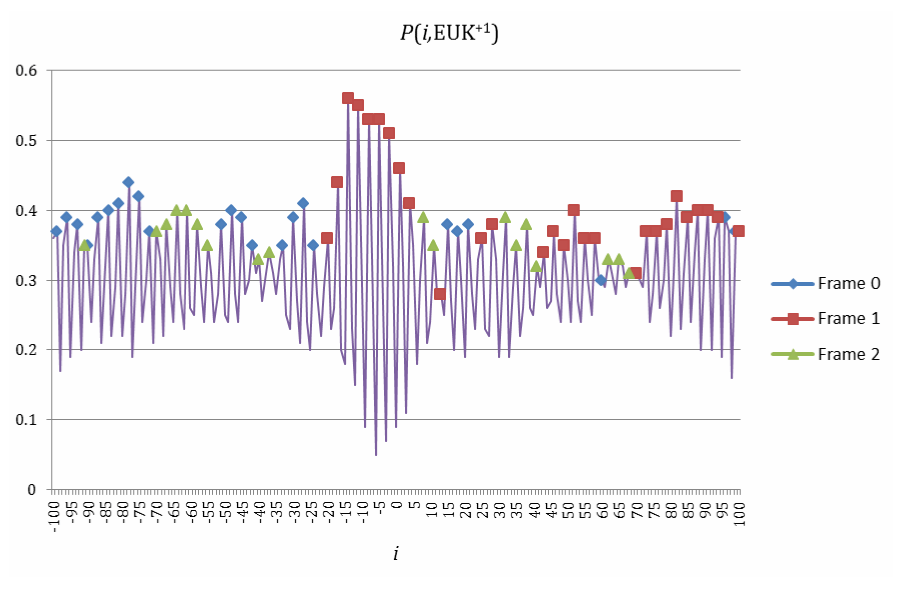Figure 6: The score function P(i, EUK+1) (Formula 2) computed on +1 frameshift of eukaryotic genes EUK+1. Before the frameshift site, the majority of local peaks (y = 18, n = 34, y/n = 53%, a = 0.565% with Formula 4) are in frame 0 (rhombuses). After the frameshift site, the majority of local peaks (y = 21, n = 34, y/n = 62%, a = 0.018% with Formula 4) are in frame 1 (squares).